## Linear Transformation

A multiplication of a matrix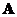with a vector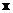will produce the transformation of the vector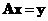. When matrixhas size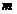by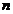and vectoris indimensions then the result is vector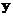indimensions.

This is the third section of Linear Algebra tutorial that you will learn about the usage of matrix and vector in solving linear equation and creating coordinate system.

The following are the topics of this section:

Matrix Generalized Inverse
Solving System of Linear Equations
Linear combination, Span & Basis Vector
Linearly Dependent & Linearly Independent
Change of basis
Matrix Rank
Matrix Range
Matrix Nullity & Null Space
Resources on Linear Algebra